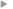## The first and second order approximations of the third-law moist-air entropy potential temperature

#### Marquet, Pascal

Année de publication
2019
Résumé
<p align=justify>It is important to be able to calculate the moist-air entropy of the atmosphere with precision. A potential temperature has already been defined from the third law of thermodynamics for this purpose. However, a doubt remains as to whether this entropy potential temperature can be represented with simple but accurate first- or second-order approximate formulas. These approximations are rigorously defined in this paper using mathematical arguments and numerical adjustments to some datasets. The differentials of these approximations lead to simple but accurate formulations for tendencies, gradients and turbulent fluxes of the moist-air entropy. Several physical consequences based on these approximations are described and can serve to better understand moist-air processes (like turbulence or diabatic forcing) or properties of certain moist-air quantities (like the static energies).</p>
Texte intégralAccès à la notice sur le site du portail documentaire de Météo-FranceListe complète des notices publiques